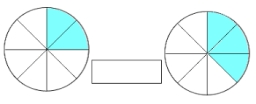# Missing number

Blank +1/6 =3/2 find the missing number

x =  1.3333

### Step-by-step explanation:

x +1/6 =3/2

6x = 8

x = 4/3 ≈ 1.333333

Our simple equation calculator calculates it.Did you find an error or inaccuracy? Feel free to write us. Thank you!Tips to related online calculators
Need help to calculate sum, simplify or multiply fractions? Try our fraction calculator.
Do you have a linear equation or system of equations and looking for its solution? Or do you have a quadratic equation?

## Related math problems and questions:

• EquationFind x: x + 1/2 = 1/3
• Fraction of a NumberIf 1/2 of 1/3 of 1/4 of 1/5 of a number is 2.5. what is the number?
• Find unknown 2Find unknown denominator: 2/3 -5/? = 1/4
• Two numbers 6Fill two natural numbers a, b: 7 + blank- blank = 5
• Unknown number 6Determine x if 1/6 of x is equal to 2/5 of the number 24.
• Reciprocal equation 3Solve reciprocal equation: 1/2 + 2/3=1/x
• Find the 24Find the difference between 2/7 and 1/21
• EquationSolve equation and check the result: 1.4x - 3/2 + x - 9,8 = x + 0,4/3 - 7 + 1,6/6
• Simplify 7Simplify. 7-1/3÷ 3-2/3 of 2+ 4-1/2÷ 2-1/4+ 1/2 solution and by step by step
• The length 6The length of 12 pipes is 10 1/2 meters. (1) find the length of one pipe (2) also find the length of 7 pipes
• Fractions and mixed numerals(a) Convert the following mixed numbers to improper fractions. i. 3 5/8 ii. 7 7/6 (b) Convert the following improper fraction to a mixed number. i. 13/4 ii. 78/5 (c) Simplify these fractions to their lowest terms. i. 36/42 ii. 27/45 2. evaluate the follow
• Simple equation 2Find X in this simple equation: X/9 = 96/108
• Divide 11Divide the product of 4 and 5/8 by 1 1/2. Write your answer as a mixed number.
• Numbers divisionWith what number should be divided mixed number 2 3/4 to get 11/12?
• LCD 2The least common denominator of 2/5, 1/2, and 3/4
• RunningJoanne ran 8 1/2 Kyleigh 1 2/3 and John 6 3/4 miles in one day. How many miles did the girls run in 3 days?
• To improper fractionChange mixed number to improper fraction a) 1 2/15 b) -2 15/17